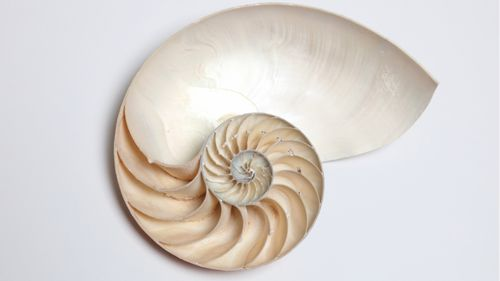Nature

# Why Does The Golden Ratio Seem To Be Everywhere In Nature?Published6CommentsThe nautilus is often used to discuss the golden spiral, but it isn't one. hilight/shutterstock

Math has a lot of special numbers but none capture the imagination quite like the golden proportion. From the Pyramids to vegetables, from Renaissance art to mollusk shells, the number is seen time and time again. And it's thought to be extremely common in nature. Except it isn’t.

There are examples that are approximations of it or have connections to the math behind it, but claiming that the golden ratio is something universal is an exaggeration. It's often just us seeing a very specific well-known pattern where there is actually a more general one.

There are two main discussion areas when it comes to the ratio in nature – Fibonacci numbers and golden spirals. Fibonacci numbers form a sequence where each number is the sum of the two preceding ones. The sequence goes like this: 1, 1, 2, 3, 5, 8, 13, 21, 34, etc. The ratio of two neighboring Fibonacci numbers is an approximation of the golden ratio. Petals and leaves are often found in this distribution, although not every plant behaves like this so we cannot claim that it's a universal property.

The golden spiral also often emerges in this argument. Both the Romanesco broccoli and the shell of the nautilus follow regular spiral structures but neither follow the traditional golden spiral. Such a spiral is created by increasing the spiral's radius by the golden proportion every 90 degrees. The shell of the nautilus, in particular, can be better described as having a spiral that expands by the golden ratio every 180 degrees. And even this is still an approximation.

If plants want to maximize the exposure of their leaves to the Sun, for example, they ideally need to grow them at non-repeating angles. Having an irrational value guarantees this, so the spirals we see in nature are a consequence of this behavior. All these distributions follow logarithmic spirals, the general mathematical form of a golden spiral.

You might consider this an Ah-ha! moment, but there are still deeper mathematical connections between all living things. What is the meaning of this? Well, the general gist is that nature is lazy and wants to do the least amount of work for the maximum result. The simplest way to do this is by giving simple instructions like “first grow, then turn a certain angle and grow again”. Mathematically this is better described by fractals, repetitive patterns that can end up producing logarithmic spirals. It is also important to remember that from the point of view of physics, spirals are low energy configurations.

So math really is the language of the universe, but it’s got a much richer vocabulary than just the golden ratio.

### ARTICLE POSTED INNature
•• golden ratio,

• golden proportion,

• nautilus,

• romanesco broccoli